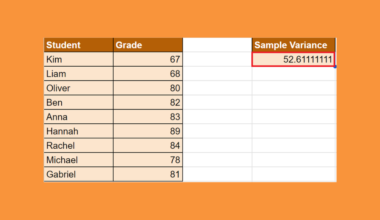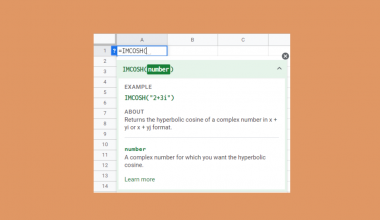# How to Use PRICEMAT Function in Google Sheets

This guide will explain how to use the PRICEMAT function in Google Sheets.

When we need to calculate the price of a security paying interest at maturity based on the expected yield, we can easily do this using the `PRICEMAT` function in Google Sheets.

The rules for using the `PRICEMAT` function in Google Sheets are the following:

• The settlement, maturity, and issue arguments must be entered using the DATE, TO_DATE, or other date parsing functions rather than by entering text.
• If we omit the day_count_convention argument, the default value is 0, which is the US (NASD) 30/360-day count convention.
• We must make sure to enter dates in the correct format YYYY-MM-DD.
•  When we input the values for the interest rate and yield, make sure to use decimal values instead of percentage values.

The `PRICEMAT` function is a powerful tool that allows us to calculate the price of a security that pays interest at maturity. For example, we can use the `PRICEMAT` function for treasury bills or a zero-coupon bond.

This function is mainly used in financial analysis to determine the value of a security. We take into account the settlement date, maturity date, issue date, annual coupon rate, yield, and day count convention.

In this guide, we will provide a step-by-step tutorial on how to use the `PRICEMAT` function in Google Sheets. Additionally, we will explore the syntax and a real example of using the function.

Great! Let’s dive right in.

## The Anatomy of the PRICEMAT Function

The syntax or the way we write the `PRICEMAT` function is as follows:

`=PRICEMAT(settlement,maturity,issue,rate,yield,[day_count_convention])`

• = the equal sign is how we start any function in Google Sheets.
• PRICEMAT() refers to our `PRICEMAT` function. This function is used to calculate the price of a security that pays interest at maturity.
• settlement is a required argument. It refers to the settlement date of the security, the date after issuance when the security is delivered to the buyer.
• maturity is also a required argument. This is the maturity or end date of the security when it can be redeemed at the face or par value.
• issue is another required argument. It refers to the date the security was initially issued.
•  rate is a required argument. This refers to the annualized rate of interest.
• yield is another required argument. It refers to the expected annual yield of the security.
• day_count_convention is an optional argument. It is an indicator of what day count method to use. By default, the value is 0, which indicates the US (NASD) 30/360 day count convention.

## The Optional Argument of the PRICEMAT Function

The `PRICEMAT` function has several required arguments for it to work properly. However, there is only one optional argument that we can utilize to apply the appropriate day count method to get accurate results.

The day_count_convention is the only optional argument of the `PRICEMAT` function, which allows us to indicate what day-count method to use in the formula.

By default, the value of the day_count_convention is 0 meaning the formula will use the US (NASD) 30/360-day count convention. This assumes that there are 30 days in a month and 360-day years as per the National Association of Securities Dealers standard.

The value 1 indicates Actual/Actual, which calculates based on the actual number of days between the specified dates and the actual number of days in the intervening years. This is often used for US Treasury Bonds and Bills, but also the most relevant for non-financial use.

If we input 2, we are indicating the Actual/360-day count convention. This calculates based on the actual number of dates between the specified dates but assumes a 360-day year.

The value 3 indicates Actual/365, which calculates based on the actual number of days between the specified dates but assumed a 365-day year.

Lastly, the value 4 indicates European 30/360. This is similar to the default 0, which calculates based on a 30-day month and a 360-day year. However, this day count convention will adjust the end-of-month dates according to the European financial conventions.

We can refer to the table below for an easier overview of the day_count_convention argument.

## A Real Example of Using PRICEMAT Function in Google Sheets

Let’s say we want to calculate the price of a security with a settlement date of March 1, 2023, a maturity date of August 1, 2023, an issue date of December 1, 2022, an annual interest rate of 3%, and an annual yield of 3.5% using the Actual/Actual day count convention.

Let’s say we want to calculate the price of a security with these data:

• settlement date of March 1, 2023
• maturity date of August 1, 2023
• issue date of December 1, 2022
• an annual interest rate of 3%
• an annual yield of 3.5% using the Actual/Actual day count convention

Our initial data set would look like this:

The spreadsheet above shows all the values we need to calculate the price of a security. We have the settlement date, the maturity date, the issue date, the interest rate, and the annual yield.

We can easily do this using the `PRICEMAT` formula below:

`=PRICEMAT(B1,B2,B3,B4,B5,1)`

In the formula above, we first selected the cell containing the settlement date, which is B1 (1/3/2023). Then, we selected the cell containing the maturity date, which is B2 (1/8/2023). Next, we added the cell containing the issue date, which is B3 (1/12/2022).

Afterward, we simply selected the cell containing the annual interest rate, which is B4 (0.03). Then, we also selected the cell containing the expected annual yield, which is B5 (0.035). Lastly, we placed 1 as our day_count_convention to calculate based on the actual/actual day count.

Our final data set would look like this:

Amazing! Now we can dive into the steps of using the `PRICEMAT` function in Google Sheets.

## How to Use PRICEMAT Function in Google Sheets

1. First, we will select an empty cell to type in our formula. To start, we will type in an equal sign and the function name. Our formula would start with “=PRICEMAT(”.

2. Then, we will simply select the cells containing the settlement date, the maturity date, and the issue date. Our formula would become “=PRICEMAT(B1,B2,B3”.

3. Next, we will select the cells containing the interest rate and the annual yield. Our formula would become “=PRICEMAT(B1,B2,B3,B4,B5”.

4. Lastly, we will choose a day count method to use. In this case, we will input “1” to calculate based on the actual number of days in the dates and intervening dates. Our final formula would be “=PRICEMAT(B1,B2,B3,B4,B5,1)”.

5. We will press the Enter key to return the result.

And tada! We have successfully used the `PRICEMAT` function in Google Sheets.

You can apply this guide whenever you need to calculate the price of a security, paying interest at maturity based on the expected yield. You can now use the `PRICEMAT` function and the various other Google Sheets formulas available to create great worksheets that work for you.

That’s pretty much it! Make sure to subscribe to our newsletter to be the first to know about the latest guides and tutorials from us.Our goal this year is to create lots of rich, bite-sized tutorials for Google Sheets users like you. If you liked this one, you'll love what we are working on! Readers receive early access to new content.

##### You May Also Like## How To Use IMCONJUGATE Function in Google Sheets

The IMCONJUGATE function in Google Sheets is useful when you want to find the complex conjugate of a…## How to Use VAR.S Function in Google Sheets

This guide will discuss how to use the VAR.S function in Google Sheets. When we need to calculate…## How to Use MAP Function in Google Sheets

This guide will discuss how to use MAPfunction in Google Sheets. When we want to map each value…## How to Normalize Data in Google Sheets

When working with datasets, it might be useful to know how to normalize data in Google Sheets. A…